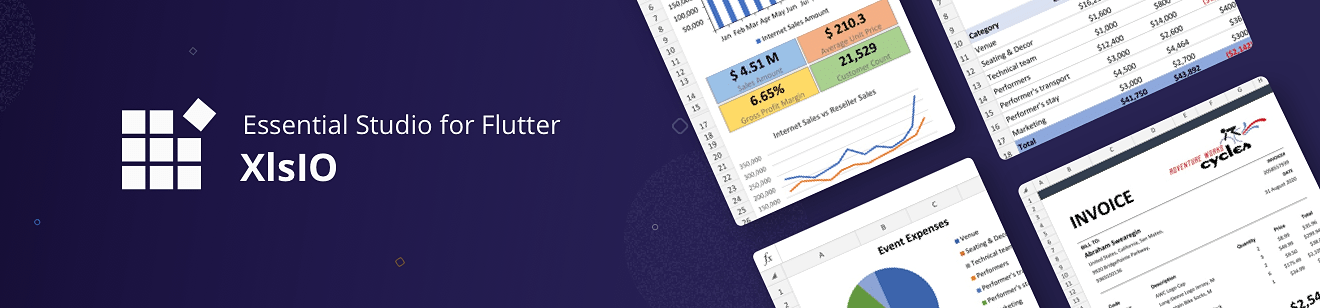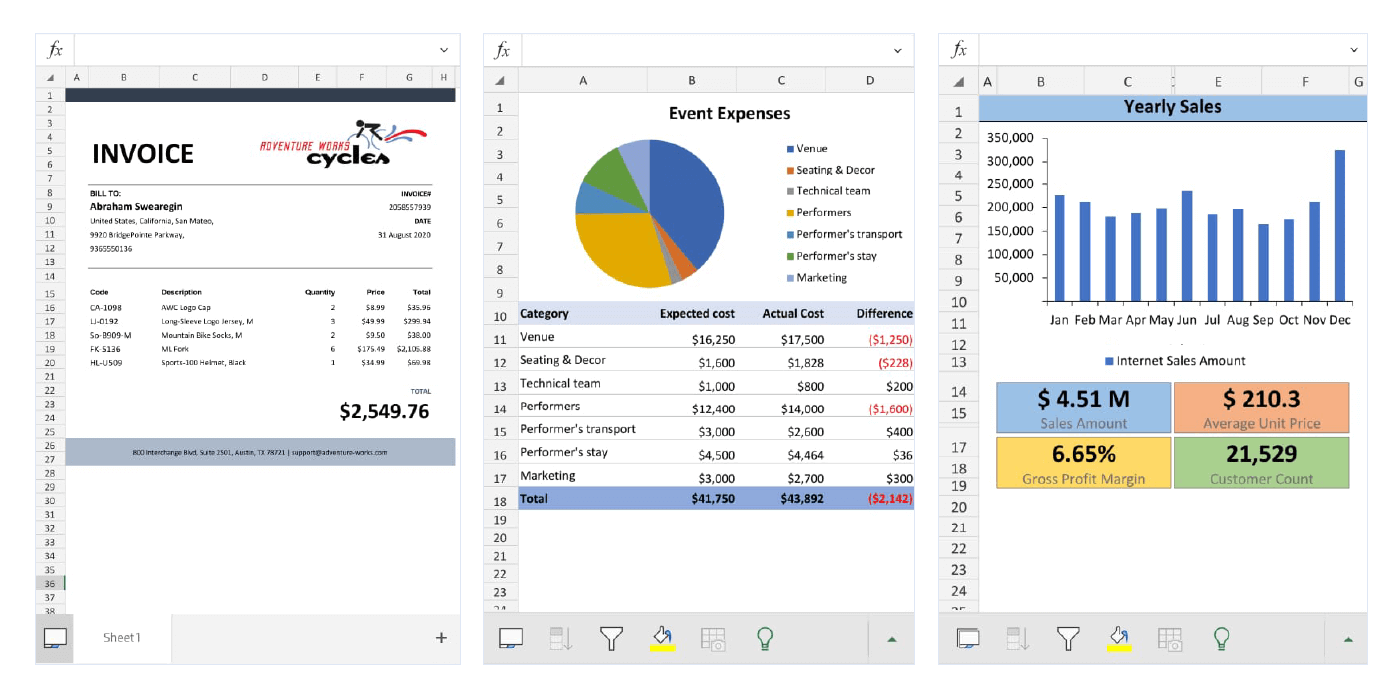# syncfusion_flutter_xlsio 19.2.51-betasyncfusion_flutter_xlsio: ^19.2.51-beta copied to clipboard

Flutter XlsIO is a Dart library for creating Excel documents with formulas, charts, images, hyperlinks, autofit rows and columns, and more.# Excel (XlsIO) Library #

Flutter XlsIO is a feature rich and high-performance non-UI Excel library written natively in Dart. It allows you to add robust Excel functionalities to Flutter applications.

## Overview #

The Excel package is a non-UI and reusable Flutter library to create Excel documents programmatically with cell values, built-in styles, cell formatting, formulas, charts, and images. The creation of Excel file are in XLSX (Excel 2007 and above) format.

Disclaimer: This is a commercial package. To use this package, you need to have either a Syncfusion Commercial License or Free Syncfusion Community license. For more details, please check the LICENSE file.## Key Features #

The following are the key features of Syncfusion Flutter XlsIO.

• Create simple Excel document in Flutter
• Apply Excel cell formatting
• Add formulas to Excel worksheet cells
• Add images to Excel worksheet
• Add charts to Excel worksheet
• Manipulate rows and columns of Excel worksheet
• Add protection to Excel document.
• Import data list to Excel Worksheet.
• Apply Excel conditional formatting.

## Get the demo application #

Explore the full capability of our Flutter widgets on your device by installing our sample browser application from the following app stores, and view sample’ codes in GitHub.

## Getting started #

Import the following package to your project to create a Excel document from scratch.

``````import 'package:syncfusion_flutter_xlsio/xlsio.dart';

``````

### Create a simple Excel document #

Add the following code to create a simple Excel document.

``````// Create a new Excel document.
final Workbook workbook = new Workbook();
//Accessing worksheet via index.
workbook.worksheets;
// Save the document.
final List<int> bytes = workbook.saveAsStream();
File('CreateExcel.xlsx').writeAsBytes(bytes);
//Dispose the workbook.
workbook.dispose();

``````

### Add text, number, and datetime values #

Use the following code to add text, number and datetime values to Excel worksheet.

``````// Create a new Excel document.
final Workbook workbook = new Workbook();
//Accessing worksheet via index.
final Worksheet sheet = workbook.worksheets;
sheet.getRangeByName('A1').setText('Hello World');
sheet.getRangeByName('A3').setNumber(44);
sheet.getRangeByName('A5').setDateTime(DateTime(2020,12,12,1,10,20));
// Save the document.
final List<int> bytes = workbook.saveAsStream();
//Dispose the workbook.
workbook.dispose();

``````

Use the following code to add formulas to Excel worksheet cells.

``````// Create a new Excel document.
final Workbook workbook = new Workbook();
//Accessing worksheet via index.
final Worksheet sheet = workbook.worksheets;
//Setting value in the cell
sheet.getRangeByName('A1').setNumber(22);
sheet.getRangeByName('A2').setNumber(44);

//Formula calculation is enabled for the sheet
sheet.enableSheetCalculations();

//Setting formula in the cell
sheet.getRangeByName('A3').setFormula('=A1+A2');

// Save the document.
final List<int> bytes = workbook.saveAsStream();
//Dispose the workbook.
workbook.dispose();

``````

### Apply formatting #

This section covers the various formatting options in a cells.

Apply GlobalStyle

Use the following code to add and apply global style to the Excel worksheet cells.

``````// Create a new Excel document.
final Workbook workbook = new Workbook();

//Accessing worksheet via index.
final Worksheet sheet = workbook.worksheets;

//Defining a global style with all properties.
//set back color by hexa decimal.
globalStyle.backColor = '#37D8E9';
//set font name.
globalStyle.fontName = 'Times New Roman';
//set font size.
globalStyle.fontSize = 20;
//set font color by hexa decimal.
globalStyle.fontColor = '#C67878';
//set font italic.
globalStyle.italic = true;
//set font bold.
globalStyle.bold = true;
//set font underline.
globalStyle.underline = true;
//set wraper text.
globalStyle.wrapText = true;
//set indent value.
globalStyle.indent = 1;
//set horizontal alignment type.
globalStyle.hAlign = HAlignType.left;
//set vertical alignment type.
globalStyle.vAlign = VAlignType.bottom;
//set text rotation.
globalStyle.rotation = 90;
//set all border line style.
globalStyle.borders.all.lineStyle = LineStyle.thick;
//set border color by hexa decimal.
globalStyle.borders.all.color = '#9954CC';
//set number format.
globalStyle.numberFormat = '_(\\$* #,##0_)';

//Apply GlobalStyle to 'A1'.
sheet.getRangeByName('A1').cellStyle = globalStyle;

//Defining Gloabl style.
//set back color by RGB value.
globalStyle.backColorRgb = Color.fromARGB(245, 22, 44, 144);
//set font color by RGB value.
globalStyle.fontColorRgb = Color.fromARGB(255, 244, 22, 44);
//set border line style.
globalStyle.borders.all.lineStyle = LineStyle.double;
//set border color by RGB value.
globalStyle.borders.all.colorRgb = Color.fromARGB(255, 44, 200, 44);

//Apply GlobalStyle to 'A4';
sheet.getRangeByName('A4').cellStyle = globalStyle;

// Save the document.
final List<int> bytes = workbook.saveAsStream();
File('ApplyGlobalStyle.xlsx').writeAsBytes(bytes);
//Dispose the workbook.
workbook.dispose();

``````

Apply Build-in Formatting

Use the following code to apply build-in style to to the Excel worksheet cells.

``````// Create a new Excel document.
final Workbook workbook = new Workbook();
//Accessing worksheet via index.
final Worksheet sheet = workbook.worksheets;

//Applying Number format.

// Save the document.
final List<int> bytes = workbook.saveAsStream();
File('ApplyBuildInStyle.xlsx').writeAsBytes(bytes);
//Dispose the workbook.
workbook.dispose();

``````

Apply NumberFormat

Use the following code to apply number format to to the Excel worksheet cells.

``````// Create a new Excel document.
final Workbook workbook = new Workbook();
//Accessing worksheet via index.
final Worksheet sheet = workbook.worksheets;

//Applying Number format.
final Range range = sheet.getRangeByName('A1');
range.setNumber(100);
range.numberFormat = '\S#,##0.00';

// Save the document.
final List<int> bytes = workbook.saveAsStream();
File('ApplyNumberFormat.xlsx').writeAsBytes(bytes);
//Dispose the workbook.
workbook.dispose();

``````

Syncfusion Flutter XlsIO supports only PNG and JPEG images. Refer to the following code to add images to Excel worksheet.

``````// Create a new Excel document.
final Workbook workbook = new Workbook();
//Accessing worksheet via index.
final Worksheet sheet = workbook.worksheets;

final Picture picture = sheet.picutes.addStream(1, 1, bytes);

// Save the document.
final List<int> bytes = workbook.saveAsStream();
//Dispose the workbook.
workbook.dispose();

``````

Import the following package to your project to create charts in Excel document from scratch.

``````import 'package:syncfusion_officechart/officechart.dart';

``````

Use the following code to add charts to Excel worksheet.

``````// Create a new Excel document.
final Workbook workbook = Workbook();
// Accessing worksheet via index.
final Worksheet sheet = workbook.worksheets;

// Setting value in the cell.
sheet.getRangeByName('A1').setText('John');
sheet.getRangeByName('A2').setText('Amy');
sheet.getRangeByName('A3').setText('Jack');
sheet.getRangeByName('A4').setText('Tiya');
sheet.getRangeByName('B1').setNumber(10);
sheet.getRangeByName('B2').setNumber(12);
sheet.getRangeByName('B3').setNumber(20);
sheet.getRangeByName('B4').setNumber(21);

// Create an instances of chart collection.
final ChartCollection charts = ChartCollection(sheet);

// Set Chart Type.
chart.chartType = ExcelChartType.column;

// Set data range in the worksheet.
chart.dataRange = sheet.getRangeByName('A1:B4');

// set charts to worksheet.
sheet.charts = charts;

// save and dispose the workbook.
final List<int> bytes = workbook.saveAsStream();
workbook.dispose();
File('Chart.xlsx').writeAsBytes(bytes);

``````

``````// Create a new Excel Document.
final Workbook workbook = Workbook();

// Accessing sheet via index.
final Worksheet sheet = workbook.worksheets;

//Creating a Hyperlink for a Website.

// Save and dispose workbook.
final List<int> bytes = workbook.saveAsStream();
workbook.dispose();

``````

### Manipulate rows and Columns #

This section covers how rows and columns are manipulated in Excel Worksheets.

Apply Autofits

Use the following code to apply autofits to single cells of the Excel worksheet.

``````// Create a new Excel Document.
final Workbook workbook = Workbook();

// Accessing sheet via index.
final Worksheet sheet = workbook.worksheets;

final Range range = sheet.getRangeByName('A1');
range.setText('WrapTextWrapTextWrapTextWrapText');
range.cellStyle.wrapText = true;

final Range range1 = sheet.getRangeByName('B1');
range1.setText('This is long text');

// AutoFit applied to a single row
sheet.autoFitRow(1);

// AutoFit applied to a single Column.
sheet.autoFitColumn(2);

// Save and dispose workbook.
final List<int> bytes = workbook.saveAsStream();
File('AutoFit.xlsx').writeAsBytes(bytes);
workbook.dispose();

``````

Use the following code to apply autofits to multiple cells of the Excel worksheet.

``````// Create a new Excel Document.
final Workbook workbook = Workbook();

// Accessing sheet via index.
final Worksheet sheet = workbook.worksheets;

// Assigning text to cells
final Range range = sheet.getRangeByName('A1:D1');
range.setText('This is Long Text');
final Range range1 = sheet.getRangeByName('A2:A5');
range1.setText('This is Long Text using AutoFit Columns and Rows');
range1.cellStyle.wrapText = true;

// Auto-Fit column the range
range.autoFitColumns();

// Auto-Fit row the range
range1.autoFitRows();

// Save and dispose workbook.
final List<int> bytes = workbook.saveAsStream();
File('AutoFits.xlsx').writeAsBytes(bytes);
workbook.dispose();

``````

Insert/Delete Rows and Colums

Use the following code to insert rows and columns to the Excel worksheet.

``````// Create a new Excel Document.
final Workbook workbook = Workbook();

// Accessing sheet via index.
final Worksheet sheet = workbook.worksheets;

Range range = sheet.getRangeByName('A1');
range.setText('Hello');

range = sheet.getRangeByName('B1');
range.setText('World');

// Insert a row
sheet.insertRow(1, 1, ExcelInsertOptions.formatAsAfter);

// Insert a column.
sheet.insertColumn(2, 1, ExcelInsertOptions.formatAsBefore);

// Save and dispose workbook.
final List<int> bytes = workbook.saveAsStream();
File('InsertRowandColumn.xlsx').writeAsBytes(bytes);
workbook.dispose();

``````

Use the following code to delete rows and columns of Excel worksheet.

``````// Create a new Excel Document.
final Workbook workbook = Workbook();

// Accessing sheet via index.
final Worksheet sheet = workbook.worksheets;

Range range = sheet.getRangeByName('A2');
range.setText('Hello');

range = sheet.getRangeByName('C2');
range.setText('World');

// Delete a row
sheet.deleteRow(1, 1);

// Delete a column.
sheet.deleteColumn(2, 1);

// Save and dispose workbook.
final List<int> bytes = workbook.saveAsStream();
File('DeleteRowandColumn.xlsx').writeAsBytes(bytes);
workbook.dispose();

``````

### Protect workbook and worksheets #

This section covers the various protection options in the Excel document.

Protect workbook elements

Use the following code to protect workbook element of Excel document.

``````// Create a new Excel Document.
final Workbook workbook = Workbook();

// Accessing sheet via index.
final Worksheet sheet = workbook.worksheets;

// Assigning text to cells
final Range range = sheet.getRangeByName('A1');
range.setText('WorkBook Protected');

final bool isProtectWindow = true;
final bool isProtectContent = true;

// Protect Workbook

// Save and dispose workbook.
final List<int> bytes = workbook.saveAsStream();
File('WorkbookProtect.xlsx').writeAsBytes(bytes);
workbook.dispose();

``````

Protect worksheet

Use the following code to protect worksheets in the Excel document.

``````// Create a new Excel Document.
final Workbook workbook = Workbook();

// Accessing sheet via index.
final Worksheet sheet = workbook.worksheets;

// Assigning text to cells
final Range range = sheet.getRangeByName('A1');
range.setText('Worksheet Protected');

// ExcelSheetProtectionOption
final ExcelSheetProtectionOption options = ExcelSheetProtectionOption();
options.all = true;

// Protecting the Worksheet by using a Password

// Save and dispose workbook.
final List<int> bytes = workbook.saveAsStream();
File('WorksheetProtect.xlsx').writeAsBytes(bytes);
workbook.dispose();

``````

### Import data #

Use the following code to import list of data into Excel Worksheet.

``````// Create a new Excel Document.
final Workbook workbook = Workbook();

// Accessing sheet via index.
final Worksheet sheet = workbook.worksheets;

//Initialize the list
final List<Object> list = [
'Toatal Income',
20000,
'On Date',
DateTime(2021, 1, 1)
];

//Import the Object list to Sheet
sheet.importList(list, 1, 1, true);

// Save and dispose workbook.
final List<int> bytes = workbook.saveAsStream();
File('ImportDataList.xlsx').writeAsBytes(bytes);
workbook.dispose();

``````

### Apply conditional formatting #

Use the following code to add and apply conditional formatting to cell or range in Excel Worksheet.

``````// Create a new Excel Document.
final Workbook workbook = Workbook();

// Accessing sheet via index.
final Worksheet sheet = workbook.worksheets;

//Applying conditional formatting to "A2".
final ConditionalFormats conditions =
sheet.getRangeByName('A2').conditionalFormats;

//Represents conditional format rule that the value in target range should be between 10 and 20
condition.formatType = ExcelCFType.cellValue;
condition.operator = ExcelComparisonOperator.between;
condition.firstFormula = '10';
condition.secondFormula = '20';
sheet.getRangeByIndex(2, 1).setText('Enter a number between 10 and 20');

//Setting format properties to be applied when the above condition is met.
//set back color by hexa decimal.
condition.backColor = '#00FFCC';
//set font color by RGB values.
condition.fontColorRgb = Color.fromARGB(255, 200, 20, 100);
//set font bold.
condition.isBold = true;
//set font italic.
condition.isItalic = true;
//set number format.
condition.numberFormat = '0.0';
//set font underline.
condition.underline = true;
//set top border line style
condition.topBorderStyle = LineStyle.thick;
// set top border color by RGB values.
condition.topBorderColorRgb = Color.fromARGB(255, 200, 1, 200);
//set bottom border line style.
condition.bottomBorderStyle = LineStyle.medium;
//set bottom border color by hexa decimal.
condition.bottomBorderColor = '#FF0000';
//set right border line style.
condition.rightBorderStyle = LineStyle.double;
// set right border color by RGB values.
condition.rightBorderColorRgb = Color.fromARGB(250, 24, 160, 200);
//set left border line style.
condition.leftBorderStyle = LineStyle.thin;
//set left border color by hexa decimal.
condition.leftBorderColor = '#AAFFAA';

//save and dispose.
final List<int> bytes = workbook.saveAsStream();
File('ConditionalFormatting.xlsx').writeAsBytes(bytes);
workbook.dispose();

``````

## Support and feedback #

• For any other queries, contact our Syncfusion support team or post the queries through the Community forums. You can also submit a feature request or a bug through our Feedback portal.
• To renew the subscription, click renew or contact our sales team at sales@syncfusion.com | Toll Free: 1-888-9 DOTNET.

Founded in 2001 and headquartered in Research Triangle Park, N.C., Syncfusion has more than 20,000 customers and more than 1 million users, including large financial institutions, Fortune 500 companies, and global IT consultancies.

Today we provide 1,000+ controls and frameworks for web (ASP.NET Core, ASP.NET MVC, ASP.NET WebForms, JavaScript, Angular, React, Vue, and Blazor), mobile (Xamarin, Flutter, UWP, and JavaScript), and desktop development (WinForms, WPF, and UWP). We provide ready-to-deploy enterprise software for dashboards, reports, data integration, and big data processing. Many customers have saved millions in licensing fees by deploying our software.

101
likes
130
pub points
92%
popularity

### Publisher

Flutter XlsIO is a Dart library for creating Excel documents with formulas, charts, images, hyperlinks, autofit rows and columns, and more.